Jan Hansen

•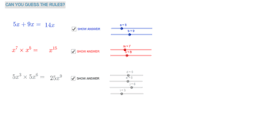Basic Algebra Rules + x

Activity

Jan Hansen

•SOLVING EQUATIONS WITH X ON BOTH SIDES

Book

Jan Hansen

•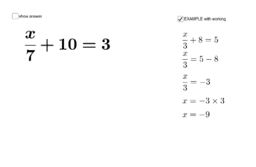asdfdddddddddddddddddddd

Activity

Jan Hansen

•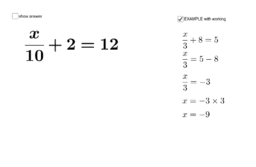ZXXXXXXXXXXXXXXXXXXXXXX

Activity

Jan Hansen

•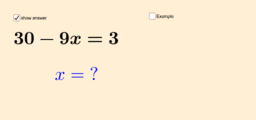fdsssssssssssssssssssssssss

Activity

Jan Hansen

•solving equations

Book

Jan Hansen

•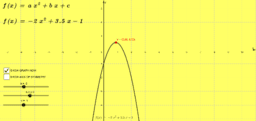Activity

Jan Hansen

•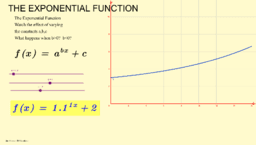The basic Exponential function x >=0

Activity

Jan Hansen

•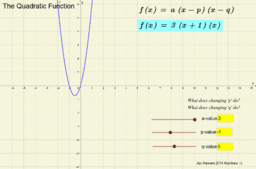Activity

Jan Hansen

•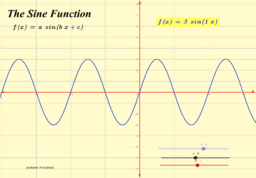The Sine Function f(x)=a sin(bx+c)

Activity

Jan Hansen

•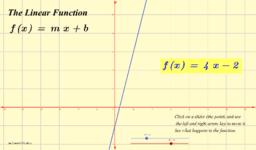The Linear Function f(x)=mx+b

Activity

Jan Hansen

•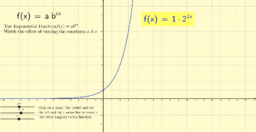Activity

Jan Hansen